Skip to content
Related Articles
Orthogonal and Orthonormal Vectors in Linear Algebra
• Last Updated : 11 May, 2020

Orthogonal Vectors: Two vectors are orthogonal to each other when their dot product is 0.
How do we define the dot product?
Dot product(scalar product) of two n-dimensional vectors A and B, is given by this expression.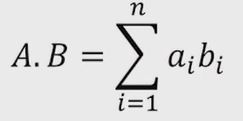Thus the vectors A and B are orthogonal to each other if and only if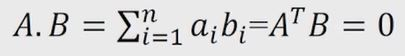Note: In a compact form the above expression can be wriiten as (A^T)B.

Example:
Consider the vectors v1 and v2 in 3D space.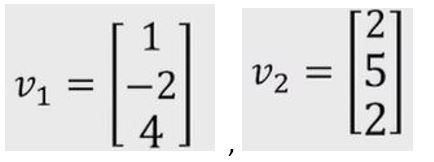Taking the dot product of the vectors.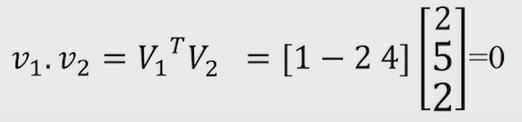Hence the vectors are orthogonal to each other.

Code: Python program to illustrate orthogonal vectors.

 `# A python program to illustrate orthogonal vector``  ` `# Import numpy module``import` `numpy``  ` `# Taking two vectors``v1 ``=` `[[``1``, ``-``2``, ``4``]]``v2 ``=` `[[``2``, ``5``, ``2``]]``  ` `# Transpose of v1``transposeOfV1 ``=` `numpy.transpose(v1)``  ` `# Matrix multiplication of both vectors``result ``=` `numpy.dot(v2, transposeOfV1)``print``(``"Result  = "``, result)``  ` `# This code is contributed by Amiya Rout`

Output:

```Result = []
```

Unit Vector:
Let’s consider a vector A. The unit vector of the vector A may be defined as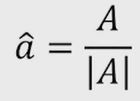Let’s understand this by taking an example. Consider a vector A in 2D space.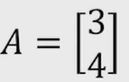The magnitude of A is given by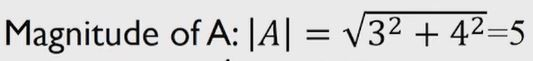So the unit vector of A can be calculated as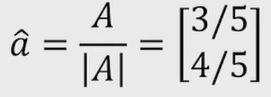Properties of unit vector:

• Unit vectors are used to define directions in a coordinate system.
• Any vectors can be written as a product of a unit vector and a scalar magnitude.

Orthonormal vectors:
These are the vectors with unit magnitude. Now, take the same 2 vectors which are orthogonal to each other and you know that when I take a dot product between these 2 vectors it is going to 0. So If we also impose the condition that we want each of these vectors to have unit magnitude then what we could possibly do is by taking this vector and then divide this vector by the magnitude of this vector as we see in unit vector. Now we can write v1 and v2 as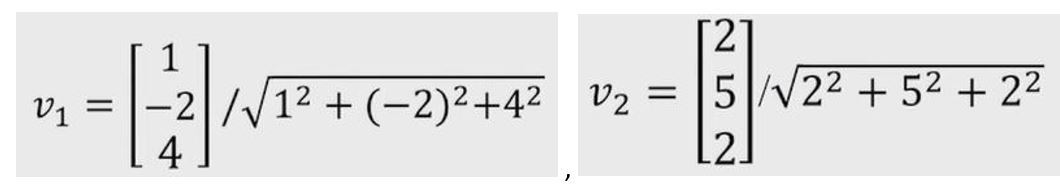So what we do is we have taken the vectors from the previous example and converted them into unit vectors by dividing them with their magnitudes. So, these vectors will still be orthogonal to each other and now individually they also have unit magnitude. Such vectors are known as orthonormal vectors.

Note: All orthonormal vectors are orthogonal by the definition itself.

Attention geek! Strengthen your foundations with the Python Programming Foundation Course and learn the basics.

To begin with, your interview preparations Enhance your Data Structures concepts with the Python DS Course. And to begin with your Machine Learning Journey, join the Machine Learning – Basic Level Course

My Personal Notes arrow_drop_up